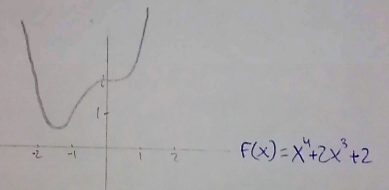#### You may also like### 2D-3D

Two circles of equal size intersect and the centre of each circle is on the circumference of the other. What is the area of the intersection? Now imagine that the diagram represents two spheres of equal volume with the centre of each sphere on the surface of the other. What is the volume of intersection?### Power Up

Show without recourse to any calculating aid that 7^{1/2} + 7^{1/3} + 7^{1/4} < 7 and 4^{1/2} + 4^{1/3} + 4^{1/4} > 4 . Sketch the graph of f(x) = x^{1/2} + x^{1/3} + x^{1/4} -x### Fractional Calculus I

You can differentiate and integrate n times but what if n is not a whole number? This generalisation of calculus was introduced and discussed on askNRICH by some school students.

# Curve Hunter

##### Age 14 to 18Challenge Level

Sanika from PSBBMS, OMR in India sent in sketches that satisfy each description:

1. A continuous curve with exactly one point with zero gradient and exactly two zeros.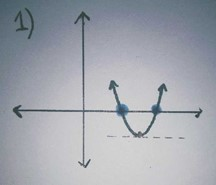2. A continuous curve with no points with zero gradient and exactly one zero.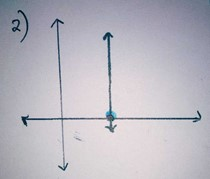3. A continuous curve with exactly one point with zero gradient and no zeros.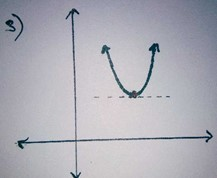4. A continuous curve with exactly two points with zero gradient and exactly three zeros.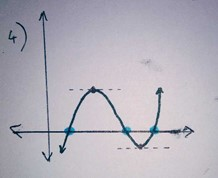5. A continuous curve with exactly two points with zero gradient and exactly two zeros.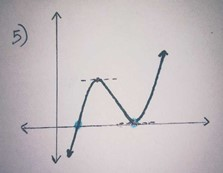6. A continuous curve with exactly one point with zero gradient and exactly one zero.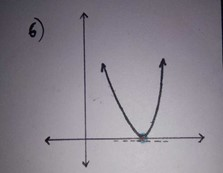7. A continuous curve with exactly two points with zero gradient and exactly one zero.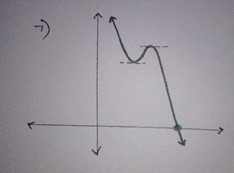8. A continuous curve with exactly two points with zero gradient and no zeros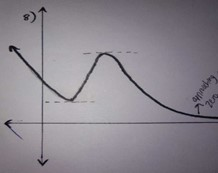Keya and Miraya from Zydus School For Excellence and Heckmondwike Grammar School also drew curves for the conditions. Keya and Miraya's graphs are creative and have the correct shapes but sometimes they haven't used the given definitions for "zeros" and "points with zero gradient". Here are their sketches: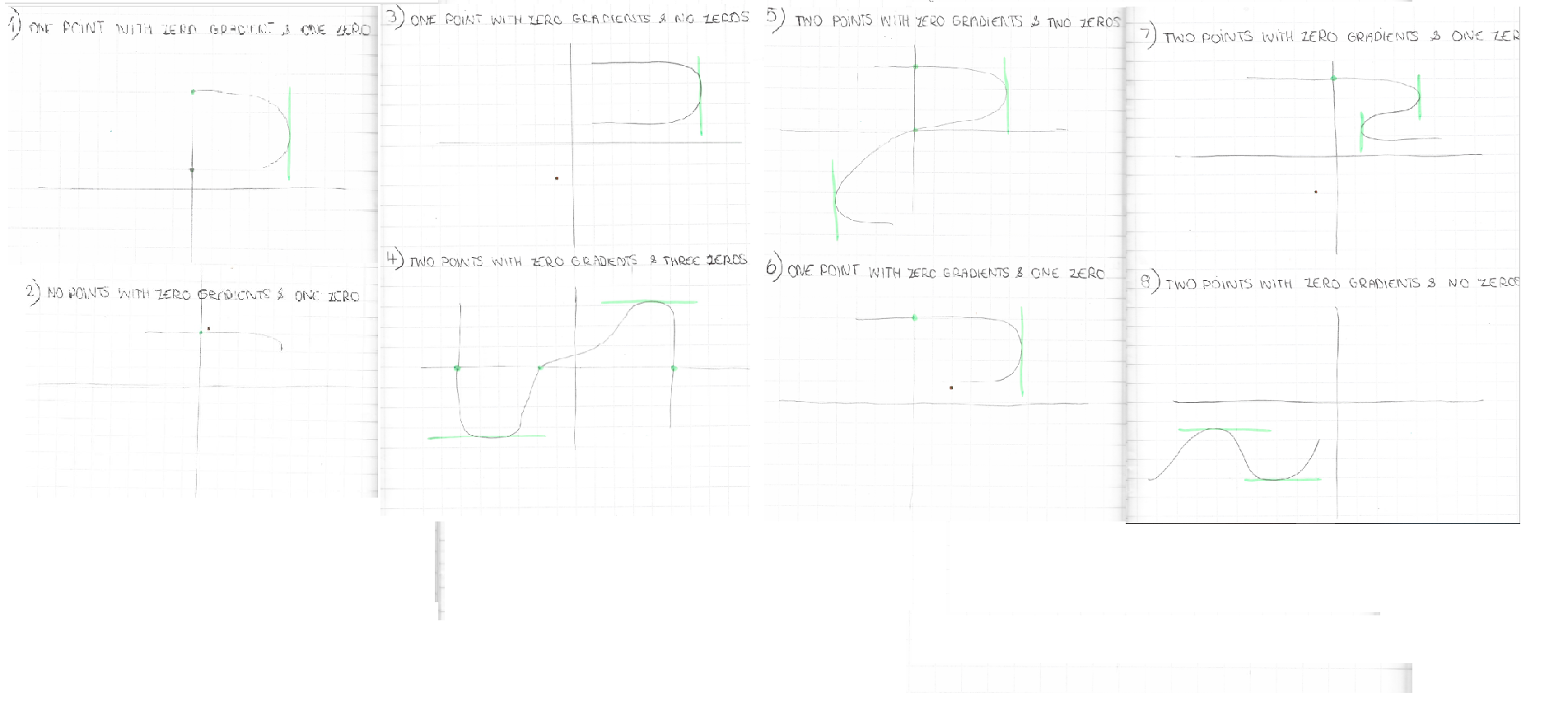By "zeros", we mean points where the curves cross the x axis, but some of Keya and Miraya's graphs include "zeros" where the curves cross the y axis.

By "points with zero gradient", we mean points where the curve is momentarily horizontal, but some of Keya and Miraya's graphs include "points with zero gradient" where the curve is momentarily vertical.

For the graphs where Keya and Miraya have confused these terms, by drawing the graphs with the same shape but the other way round with respect to the x- and y- axes, the graphs would meet the conditions with the given definitions of "zeros" and "points with zero gradient".

Some of Keya and Miraya's curves end in the middle of the graph. This means they only exist for certain values of $x.$ For example for condition 8, it looks like their curve is only defined for $x\leq-1.$ If that is the case, then their curve is continuous for $x\leq-1,$ and so if we only consider those values of $x,$ then it satisfies the condition. However, the other mathematicians who submitted solutions usually assumed that the curves had to be continuous for all values of $x.$

Another interesting difference between Keya and Miraya's graphs and the other graphs submitted is that some of Keya and Miraya's graphs go from right to left as well as left to right, or in other words some of them have more than one y-coordinate corresponding to the same x-coordinate. This doesn't mean they are not continuous curves, but it does mean they can't be called "functions". Mathematicians often prefer to work with "functions" - that is, curves with only one y-coordinate for each x-coordinate (but possibly more than one x-coordinate for single y-coordinates).

Is there a number of zeros and a number of points with zero gradient for which a continuous curve cannot be drawn? Or is it possible to draw a continuous curve with any number of zeros and any number of points with zero gradient?

Keya and Miraya said that it is possible to draw a continuous curve with any number of zeros and any number of points with zero gradient, and they are right in that using their definitions, it would be.

Using the definitions given and the assumptions about curves not ending in the middle of the graph, Sanika gave this answer, which is also correct:

It is not possible to draw a continuous graph with any number of roots and points with zero gradients. For instance, if the number of roots is 2 or more than the number of points with a gradient of zero, then you can't draw a graph fulfilling all the conditions.

Sarah from LAE Tottenham in the UK explained the same idea using algebra and examples: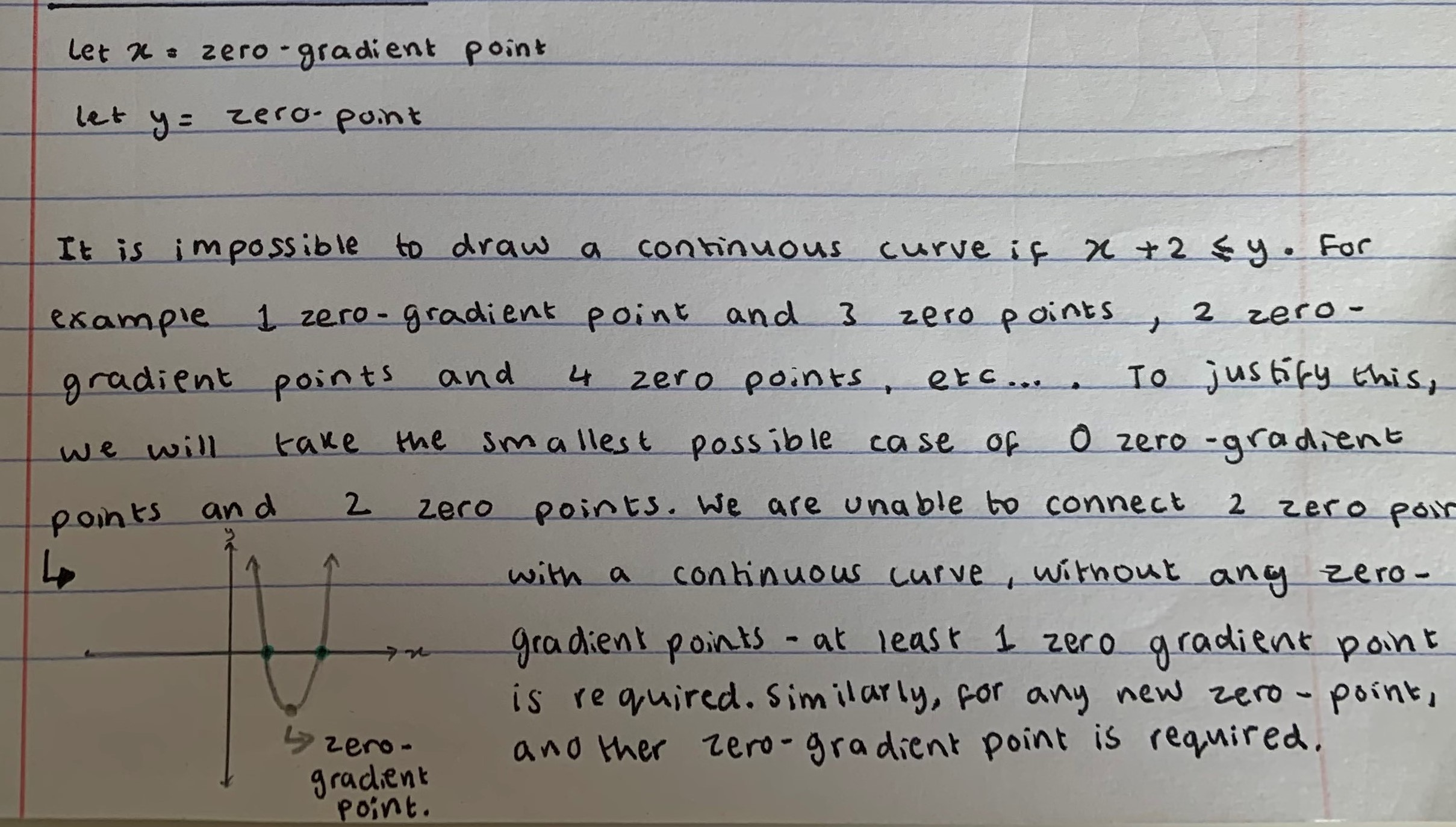David from IES Maximo Laguna in Spain wrote exactly when it is possible: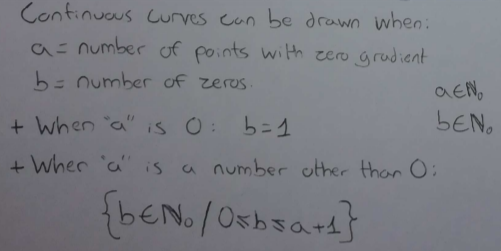Can you give examples of algebraic equations that satisfy each of the conditions above?

Dr Barker's year 12 class from Jersey College for Girls, Mahdi from Mahatma Gandhi International School in India and David all sent in an equation and its graph for each condition, and Sanika found an equation for each condition. Click to see the examples for each one.

1. A continuous curve with exactly one point with zero gradient and exactly two zeros.

Mahdi: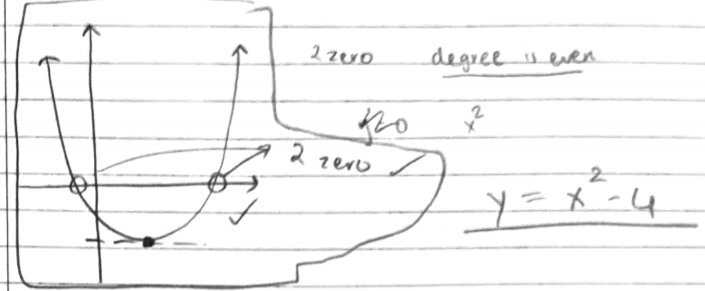(note that Mahdi's equation does not perfectly match Mahdi's sketch)

Dr Barker's class:

We graphed a parabola with two separate roots, which gave us one point of zero gradient (turning point) and two zeros (roots).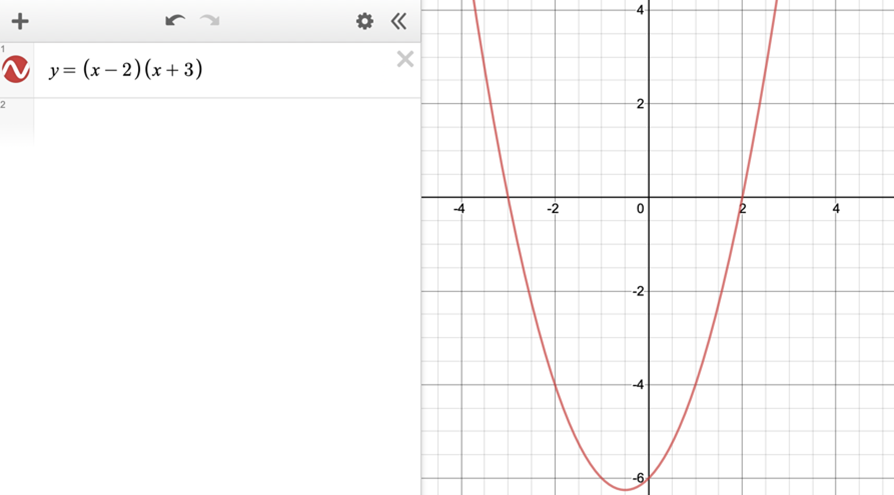David: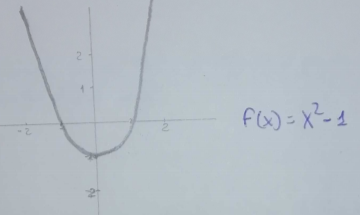Sanika:
$x^2-1=y$

2. A continuous curve with no points with zero gradient and exactly one zero.

Dr Barker's class:
We graphed a cubic function which passes through the x axis once and has a point of inflection- so no points of zero gradient.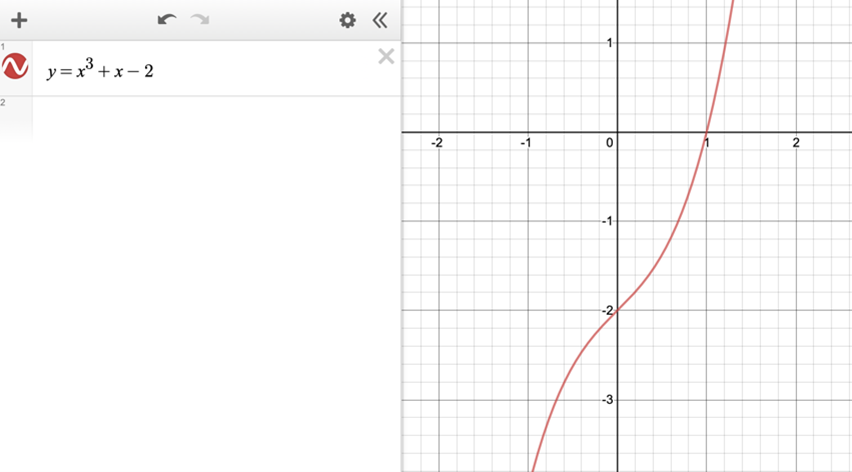Mahdi: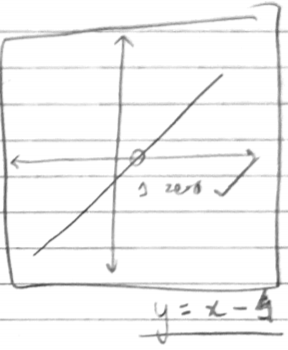David: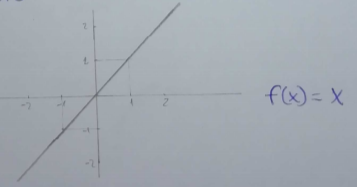Sanika:
$x=1$

3. A continuous curve with exactly one point with zero gradient and no zeros.

Dr Barker's class:
We graphed a parabola with no roots which gave one point of zero gradient.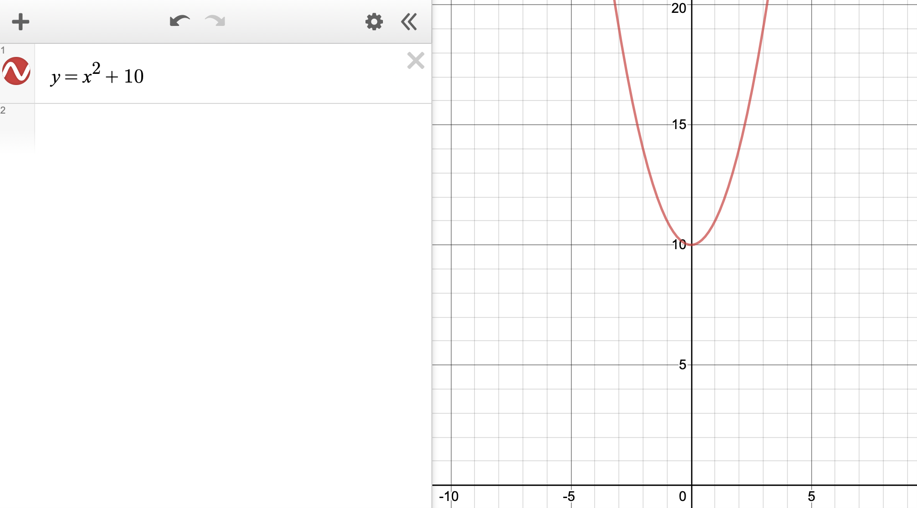Mahdi: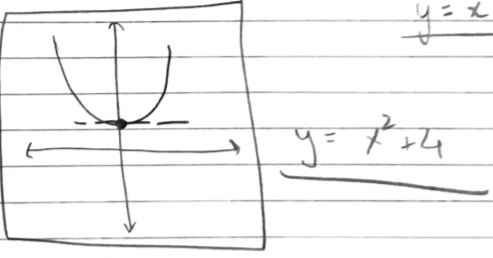David: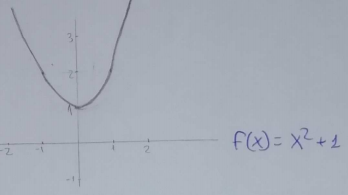Sanika:
$x^2-x+1=y$

4. A continuous curve with exactly two points with zero gradient and exactly three zeros.

Dr Barker's class:
We graphed a cubic with 3 separate roots (3 zeros) and 2 turning points (2 points of zero gradient).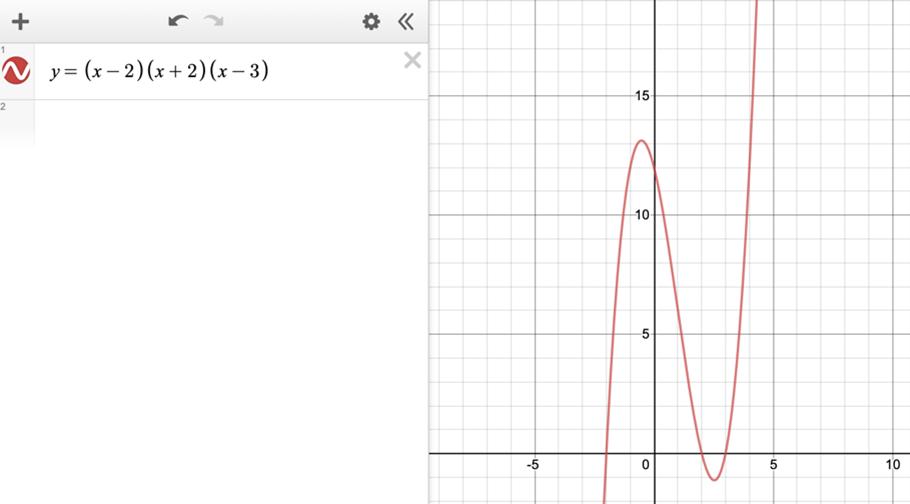Mahdi: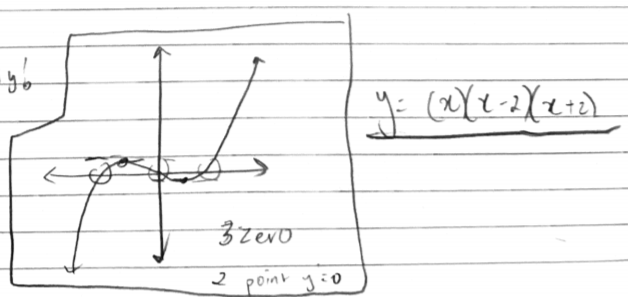David: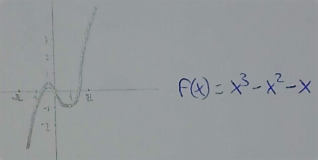Sanika:
$x^3 + 6x^2 + 11x + 6 = y$

5. A continuous curve with exactly two points with zero gradient and exactly two zeros.

Dr Barker's class:
We graphed a cubic with two roots (one repeated root so there are 2 zeros) and two turning points (two points of zero gradient).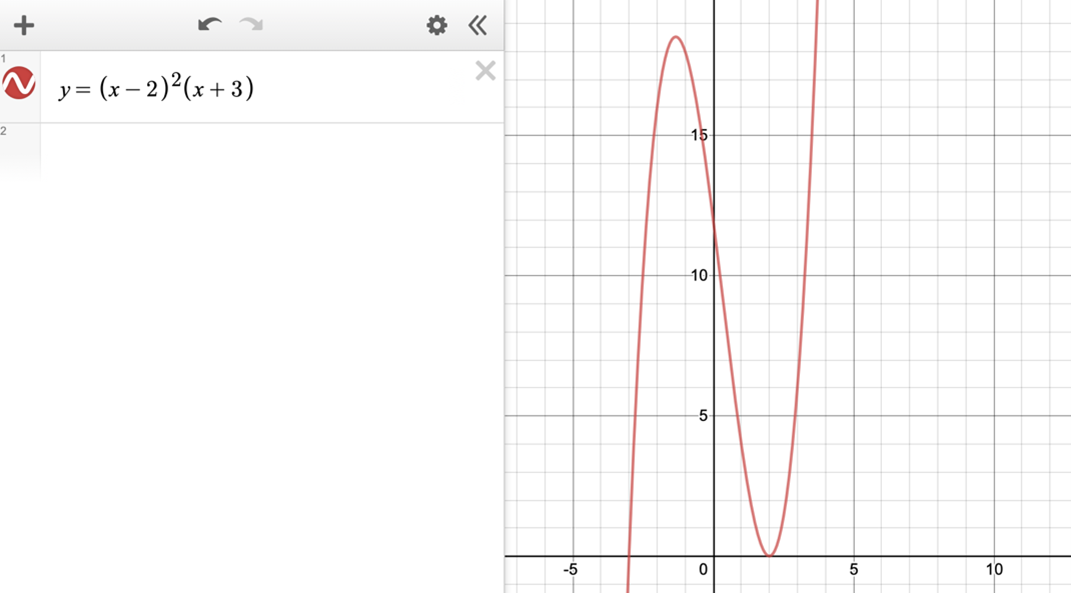Mahdi: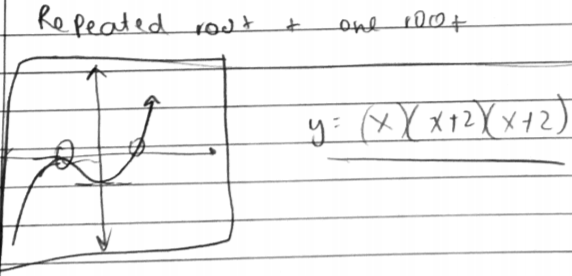David: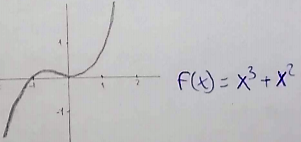Sanika:
$x^3 + 4x^2 + 5x + 2 = y$

6. A continuous curve with exactly one point with zero gradient and exactly one zero.

Dr Barker's class:
We graphed a parabola with one repeated root (one zero and one point of zero gradient).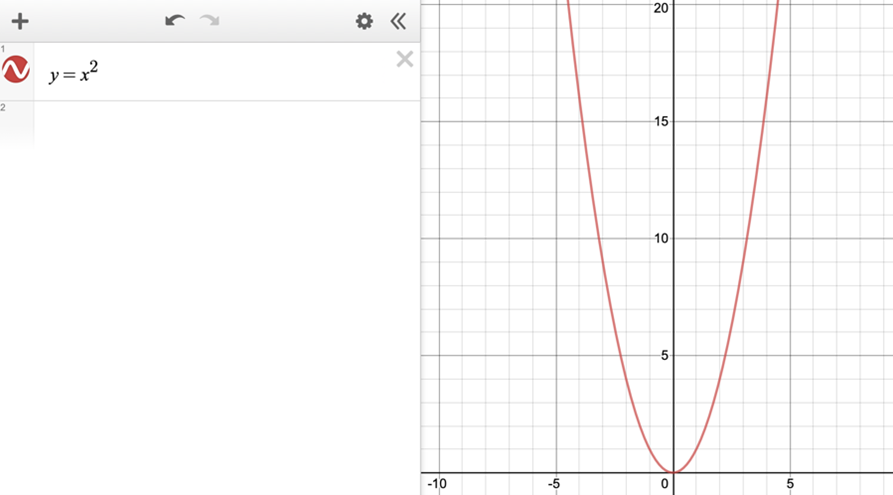Sanika:
$x^2 + 2x + 1 = y$

Mahdi: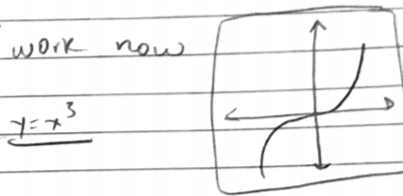David: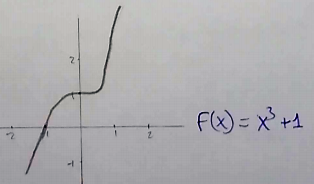7. A continuous curve with exactly two points with zero gradient and exactly one zero.

Dr Barker's class's curve actually satisfies condition 6, not condition 7:
We graphed a cubic with one stationary point (one point of zero gradient) and one intersection with the x axis (one zero).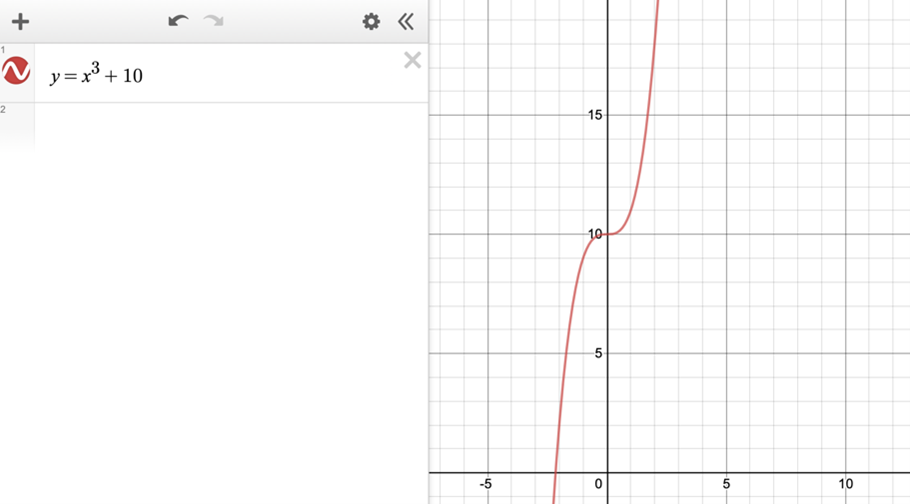Mahdi: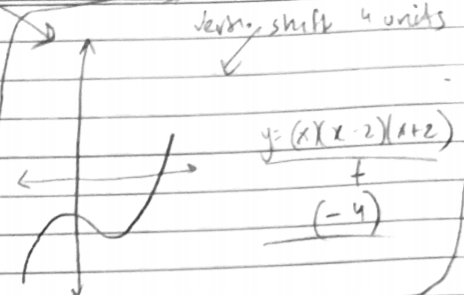David: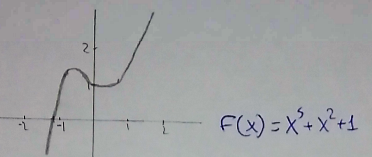Sanika:

$x^3+2x^2+10=y$
These cubics do satisfy condition 7.

8. A continuous curve with exactly two points with zero gradient and no zeros.

Dr Barker's class:
We graphed a quartic function, with two turning points (2 points of zero gradient) and no intersection with the x axis (no zeros).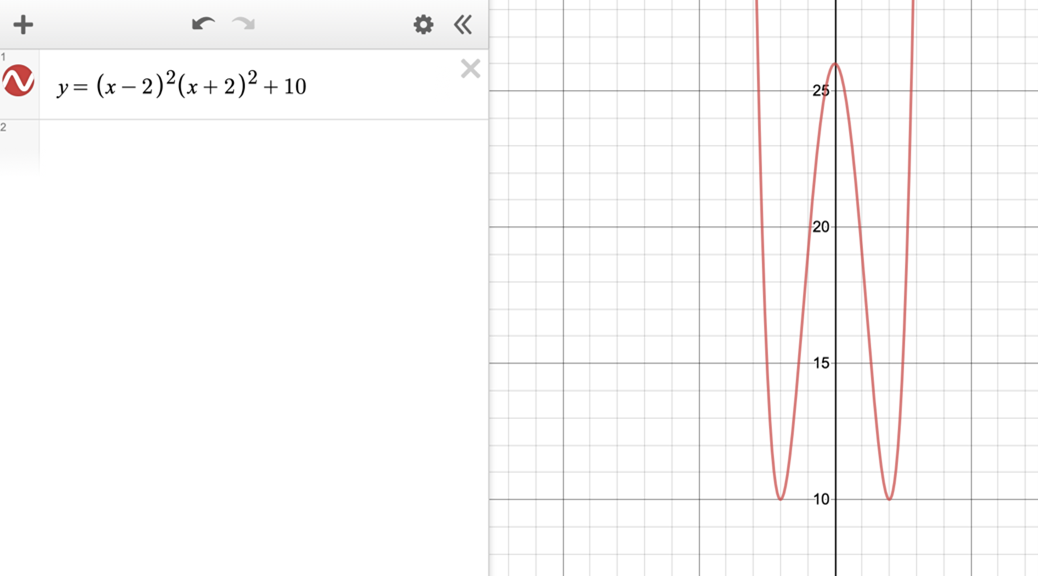Actually, this quartic has three points of zero gradient, but Mahdi's and David's have two.

Mahdi: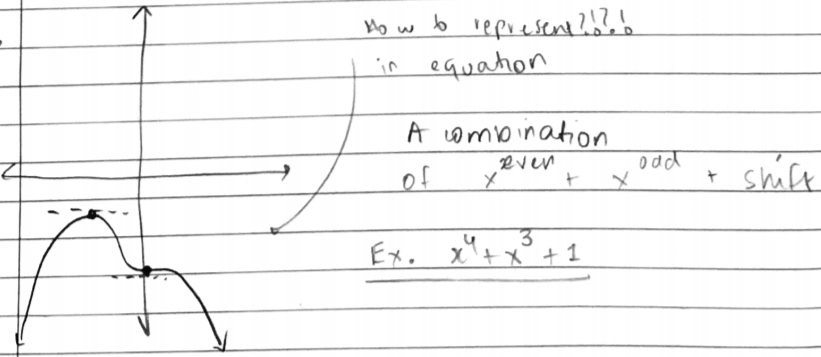This is the graph of Mahdi's final equation: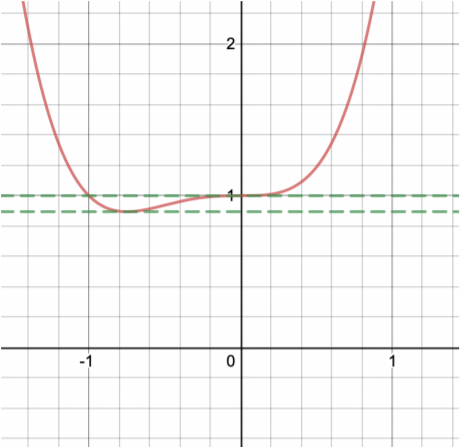David: